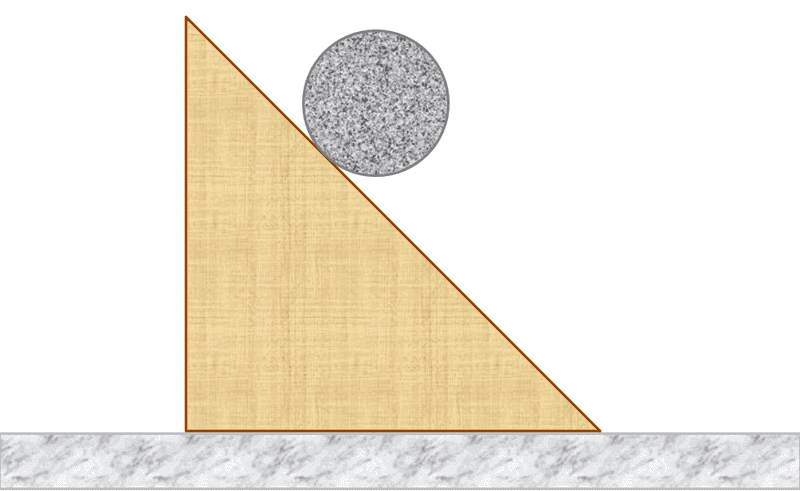# Acceleration, Uniform Ball on Incline

• Phys student
In summary, The system consists of a solid ball of mass m and a right angled wedge of mass M and angle θ from the horizontal. The ball rolls without slipping down the wedge, which itself can slide without friction on a horizontal floor. To find the acceleration of the ball relative to the wedge, we can use generalized coordinates and take the derivative of the total energy, which is the sum of kinetic energy and potential energy. By relating the coordinates of the ball and wedge, we can solve for the acceleration of the ball, which is given by the expression: \ddot{ξ} =\frac{10gM^2sin{θ}}{5m^2+5m(m-M)\cos(2θ)-

## Homework Statement[/B]
A uniform solid ball of mass $m$ rolls without slipping down a right angled wedge of mass $M$ and angle $θ$ from the horizontal, which itself can slide without friction on a horizontal floor. Find the acceleration of the ball relative to the wedge.
2. The attempt at a solution
Attempt using generalized coordinates:
Let us denote the coordinate of the ball as $ξ$ (which is along the incline) and the coordinate of the wedge as $η$. The kinetic energy of the system is:

$$K=\frac{1}{2}m\dotξ^2+\frac{1}{2}Iω^2+\frac{1}{2}M\dotη^2=\frac{1}{2}m\dotξ^2+\frac{2}{10}m\dotξ^2+\frac{1}{2}M\dotη^2=\frac{7}{10}m\dotξ^2+\frac{1}{2}M\dotη^2$$
And the change in potential energy is:
$$V=-mgξ\sin{θ}$$
The sum of both energies is the total energy, which is constant in time. We take the derivative of it:
$$\dot{E}=\frac{d}{dt}E=\frac{d}{dt} K+V=\frac{d}{dt} (\frac{7}{10}m\dotξ^2+\frac{1}{2}M\dotη^2-mgξ\sin{θ})=\frac{7}{5}m\dotξ\ddotξ+M\dotη\ddotη-mg\dotξ\sin{θ}=0$$
Finally, we need to relate both coordinates. Since the center of mass of the system doesn't move we have:
$$mξ\cos(θ)=Mη$$ $$\frac{mξ\cos(θ)}{M}=η$$

We substitute $η$ in our derivative and we get:
$$\dot{E}=\frac{7}{5}m\dotξ\ddotξ+\frac{m^2\dot{ξ}\ddot{ξ}\cos^2{θ}}{M}-mg\dot{ξ}\sin{θ}=0$$
We solve for $\ddotξ$:
$$\ddotξ =\frac{5Mg\sin{θ}}{7M+5m\cos^2{θ}}$$
Can someone please confirm if this solution is correct?

Last edited:
Phys student said:
Let us denote the coordinate of the ball as $ξ$ (which is along the incline) and the coordinate of the wedge as $η$. The kinetic energy of the system is:

$$K=\frac{1}{2}m\dotξ^2+\frac{1}{2}Iω^2+\frac{1}{2}M\dotη^2=\frac{1}{2}m\dotξ^2+\frac{2}{10}m\dotξ^2+\frac{1}{2}M\dotη^2=\frac{7}{10}m\dotξ^2+\frac{1}{2}M\dotη^2$$
And the change in potential energy is:
$$V=-mgξ\sin{θ}$$
Not exactly sure how you're defining ξ. The potential energy expression implies it's along the slope, relative to the wedge, but the KE expression implies it is in the instantaneous direction of linear movement of the ball relative to the ground.

haruspex said:
Not exactly sure how you're defining ξ. The potential energy expression implies it's along the slope, relative to the wedge, but the KE expression implies it is in the instantaneous direction of linear movement of the ball relative to the ground.
It is along the slope, How did the kinetic energy expression make you assume it's relative to the ground?

Phys student said:
It is along the slope, How did the kinetic energy expression make you assume it's relative to the ground?
##\frac{1}{2}m\dotξ^2##

haruspex said:
##\frac{1}{2}m\dotξ^2##
So the expression should have been: $\frac{1}{2}m({{\vec{\dotξ}}}+{{\vec{\dotη}}})^2$?

Last edited:
Phys student said:
So the expression should have been: $\frac{1}{2}m({{\vec{\dotξ}}}+{{\vec{\dotη}}})^2$?

Is the kinetic energy of the ball the sum of the kinetic energies of both coordinates? (ball moving with respect to the incline and the ground)

$$\frac{1}{2}m\dotξ^2 +\frac{1}{2}m\dotη^2$$

Last edited:
Phys student said:
Is the kinetic energy of the ball the sum of the kinetic energies of both coordinates? (ball moving with respect to the incline and the ground)

$$\frac{1}{2}m\dotξ^2 +\frac{1}{2}m\dotη^2$$

No.The translational KE of the ball is 1/2 m(vx2+vy2), vx and vy being the horizontal and vertical velocity components with respect to the ground. Express them with the velocity of the wedge and the velocity of the ball with respect to the wedge. Note that ξ has both horizontal and vertical components.

ehild said:
No.The translational KE of the ball is 1/2 m(vx2+vy2), vx and vy being the horizontal and vertical velocity components with respect to the ground. Express them with the velocity of the wedge and the velocity of the ball with respect to the wedge. Note that ξ has both horizontal and vertical components.

The $x$ component of the ball's velocity with respect to the ground is:
$$v_x= \dot{ξ}\cos{θ}-\dot{η}=\dot{ξ}\cos{θ}(1-\frac{m}{M})$$
And the $y$ component is:
$$v_y =\dot{ξ}\sin{θ}$$
Right?

Phys student said:
The $x$ component of the ball's velocity with respect to the ground is:
$$v_x= \dot{ξ}\cos{θ}-\dot{η}=\dot{ξ}\cos{θ}(1-\frac{m}{M})$$
And the $y$ component is:
$$v_y =\dot{ξ}\sin{θ}$$
Right?
Yes.

ehild said:
Yes.
What about the rotational kinetic energy? What $v$ do you use?

The ball rotates with angular frequency ω around the CM, it is independent from the velocity of the wedge.

I believe you are overdoing things.
Try calculating total energy at the start of the system, and then calculate at the end of the system.
Find out an equation for velocity, use Newton's third law of motion. You'll get what you are looking for.

ehild said:
The ball rotates with angular frequency ω around the CM, it is independent from the velocity of the wedge.
So in the end we have:

$$K=\frac{1}{2}m\dot{ξ}^2(\sin^2{θ}+\cos^2{θ}(1-\frac{m}{M})^2)+\frac{2}{10}m\dot{ξ}^2 +\frac{m^2\cos^2{θ}\dot{ξ}^2}{2M}$$
$$V=-mgξ\sin(θ)$$
$$\dot{E}=m\ddot{ξ}(\sin^2{θ}+\cos^2{θ}(1-\frac{m}{M})^2)+\frac{4}{10}m\ddot{ξ}+\frac{m^2\cos^2{θ}\ddot{ξ}}{M}-mg\sin{θ}=0$$
When we solve for $\ddot{ξ}$ we get this expression:
$$\frac{10gM^2sin{θ}}{5m^2+5m(m-M)\cos(2θ)-5mM+14M^2}$$

You can factor out ##(\dot \xi )^2## and simplify the expression for K. E=K+V =0. ##\dot E## is not what you wrote, but the equation ##
m\ddot{ξ}(\sin^2{θ}+\cos^2{θ}(1-\frac{m}{M})^2)+\frac{4}{10}m\ddot{ξ}+\frac{m^2\cos^2{θ}\ddot{ξ}}{M}-mg\sin{θ}=0
##
is correct. You madethe final expression too complicated, simplify. No need to introduce cos(2θ).